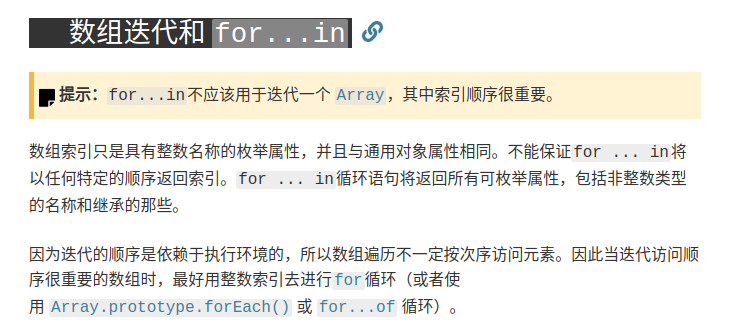## 一、数组基本遍历

### 1.for…in

for…in其实是对象的遍历方式，并不是数组专有，使用for…in将循环遍历对象本身的所有可枚举属性，以及对象从其构造函数原型中继承的属性，其遍历顺序与Object.keys()函数取到的列表一致。

``````let list = [7, 5, 2, 3]
list = 1
list['a'] = 1
console.log(JSON.stringify(Object.keys(list)))

for (let key in list) {
console.log(key, list[key])
}
``````

``````> ["0","1","2","3","10","a"]
> 0, 7
> 1, 5
> 2, 2
> 3, 3
> 10, 1
> a, 1
``````### 2.for…of

``````let list = [7, 5, 2, 3]
list = 4
list = 5
list = 1
// 此时下标6、7、8、9为空元素
list['a'] = 'a'

for (let value of list) {
console.log(value)
}

``````

``````> 7
> 5
> 2
> 3
> 5
> 4
>   //　遍历空元素
> 　//　遍历空元素
> 　//　遍历空元素
> 　//　遍历空元素
> 1
``````

### 3.取数组长度进行遍历

``````let list = ['a', 'b', 'c', 'd']
list = 'e'
list = 'z'
list['a'] = 0

for (let idx = 0; idx < list.length; idx++) {
console.log(idx, list[idx])
}
``````

``````> 0, a
> 1, b
> 2, c
> 3, d
> 4, e
> 5, //空元素
> 6,
> 7,
> 8,
> 9,
> 10, z
``````

### 4.forEach遍历

forEach是数组的一个高阶函数,用法如下:

``````arr.forEach(callback[, thisArg])
``````

callback

• currentValue
数组中正在处理的当前元素。
• index 可选
数组中正在处理的当前元素的索引。
• array 可选
forEach() 方法正在操作的数组。

thisArg可选

forEach遍历数组会按照数组下标升序遍历，并且会忽略空元素：

``````let list = ['a', 'b', 'c', 'd']
list = 'e'
list = 'z'
list['a'] = 0

list.forEach((value, key, list) => {
console.log(key, value)
})
``````

``````> 0, a
> 1, b
> 2, c
> 3, d
> 4, e
> 10, z
``````

#### for…of和forEach遍历中删除元素

``````let list = ['a', 'b', 'c', 'd']

for (let item of list) {
if (item === 'a') {
list.splice(0, 1)
}
console.log(item)
}
``````

``````> a
> c
> d
``````

forEach遍历中删除元素：

``````let list = ['a', 'b', 'c', 'd']

list.forEach((item, idx) => {
if (item === 'a') {
list.splice(0, 1)
}
console.log(item)
})
``````

``````> a
> c
> d
``````

#### for…of和forEach遍历中增加元素

for…of遍历中增加元素：

``````let list = ['a', 'b', 'c', 'd']
for (let item of list) {
if (item === 'a') {
list.splice(1, 0, 'e')
}
console.log(item)
}
``````

``````> a
> e
> b
> c
> d
``````

forEach遍历中增加元素：

``````let list = ['a', 'b', 'c', 'd']

list.forEach((item, idx) => {
if (item === 'a') {
list.splice(1, 0, 'e')
}
console.log(item)
})
``````

``````> a
> e
> b
> c
``````

## 二、利用高阶函数遍历数组

### 1. map

map() 方法参数与forEach完全相同，二者区别仅仅在于map会将回调函数的返回值收集起来产生一个新数组。

``````let list = [1, 2, 3, 4]
let result = list.map((value, idx) => value * 2)
console.log(result)　//　输出[2,4,6,8]
``````

### 2.filter

filter() 参数与forEach完全一致，不过它的callback函数应该返回一个真值或假值。filter() 方法创建一个新数组, 新数组包含所有使得callback返回值为真值（Truthy，与true有区别）的元素。

``````let list = [1, 2, 3, 4]
let result = list.filter((value, idx) => value % 2 === 0)
console.log(result) // 输出［2,4］
``````

### 3. find/findIndex

find() 方法返回数组中使callback返回值为Truthy的第一个元素的值，没有则返回undefined。使用非常简单，比如找出数组中第一个偶数：

``````let list = ['1', '2', '3', '4']
let result = list.find(value => value % 2 === 0)
console.log(result) // 输出 2
``````

findIndex()方法与find方法很类似,只不过findIndex返回使callback返回值为Truthy的第一个元素的索引，没有符合元素则返回-1。比如找出数组中第一个偶数的下标：

``````let list = [1, 2, 3, 4]
let result = list.findIndex(value => value % 2 === 0)
console.log(result)　// 输出 1
``````

### 4.every/some

``````let list = [1, 2, 3, 4]
// 判断数组中是否每个元素小于10
let result = list.every(value => {
return value < 10
})
console.log(result) // 输出true

//　判断是否每个元素大于2
result = list.every(value => {
return value > 2
})
console.log(result) // 输出false

// 判断是数组中否存在1
result = list.some(value => {
return value === 1
})
console.log(result) // 输出true

// 判断数组中是否存在大于10的数
result = list.some(value => {
return value > 10
})
console.log(result) // 输出false
``````

### 5.reduce/reduceRight 累加器

callback

• accumulator
累计器累计回调的返回值; 它是上一次调用回调时返回的累积值，或initialValue（见于下方）。

• currentValue
数组中正在处理的元素。

• currentIndex 可选
数组中正在处理的当前元素的索引。 如果提供了initialValue，则起始索引号为0，否则为1。

• array 可选
调用reduce()的数组

initialValue可选

reduce() 方法对数组中的每个元素执行一个由您提供的reducer函数(升序执行)，将其结果汇总为单个返回值，而reduceRight只是遍历顺序相反而已。

``````let list = [
{
id: 1,
parentId: ''
},
{
id: 2,
parentId: ''
},
{
id: 3,
parentId: 1
},
{
id: 4,
parentId: 2,
},
{
id: 5,
parentId: 3
},
{
id: 6,
parentId: 3
}
]
``````

``````
[
{
"id":1,
"parentId":"",
"children":[
{
"id":3,
"parentId":1,
"children":[
{
"id":5,
"parentId":3
},
{
"id":6,
"parentId":3
}
]
}
]
},
{
"id":2,
"parentId":"",
"children":[
{
"id":4,
"parentId":2
}
]
}
]
``````

``````function listToTree(srcList) {
let result = []
// reduce收集所有节点信息存放在对象中，可以用forEach改写，不过代码会多几行
let nodeInfo = list.reduce((data, node) => (data[node.id] = node, data), {})

// forEach给所有元素找妈妈
srcList.forEach(node => {
if (!node.parentId) {
result.push(node)
return
}
let parent = nodeInfo[node.parentId]
parent.children = parent.children || []
parent.children.push(node)
})
return result
}
``````

¥赞赏